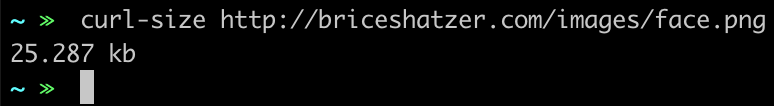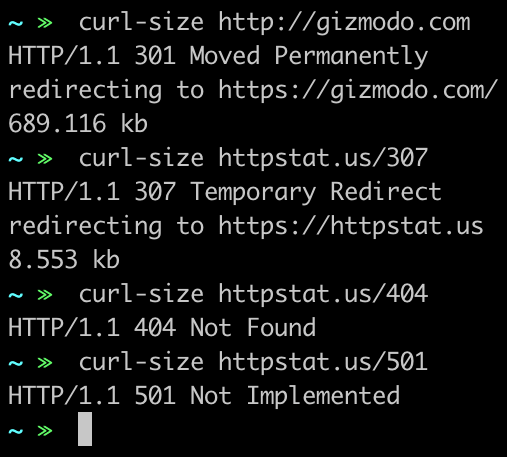April 25, 2019

## Check a File's Size With This Simple Command-line Function

I recently had a project dealing with image compression/hosting and I wanted a way to check the size of a hosted file that was quicker than digging into the network panel or opening Postman each time.

So, I wrote a simple function that I’ve added to my .bashrc file to do exactly that.

Basically it is just a simple wrapper around curl that:

1. Uses `curl -I` to fetch the header of a user provided URL
2. Checks to make sure the header returned useful information
3. Takes that useful header information and formats it in a way that is easy to understand.

### Code

``````
function curl-size {
if [[ -z \$1 ]]; then
printf '%s\n' "Please provide a URL"
else
response=\$(curl -sI "\$1" | tr -d '\r' )
statuscode=\$(echo \$response | awk '/^HTTP/ {print}')
bytelength=\$(echo \$response | awk '/[cC]ontent-[lL]ength/ {print \$2}')

if [[ \$statuscode != *"200"* ]]; then
print \$statuscode
fi

function _doMath {
divisor=\$1
print "scale=3;\$bytelength/\$divisor" | bc -l
}

if [[ -z \$bytelength || (\$bytelength == "0") ]]; then
value=""
elif ((\$bytelength>1000000000));then #1*10^9
value=\$(_doMath 1000000000)
unit="gb"
elif ((\$bytelength>1000000));then #1*10^6
value=\$(_doMath 1000000)
unit="mb"
elif ((\$bytelength>1000));then
value=\$(_doMath 1000)
unit="kb"
else
value="\$bytelength"
unit="bytes"
fi
printf '%s\n' "\$(printf '%s\n' "\$value" | grep -o '.*[1-9]') \$unit"
fi
}

``````

### In Use

Using it for it’s original purpose…It even handles URLs that return non-200 status codes…« All Posts